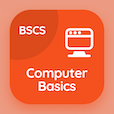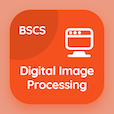Management Online Courses (MBA)

MBA Statistics MCQ Questions

MBA Statistics MCQs PDF - Chapters

# Multiple Regression Model Multiple Choice Questions Online p. 1

Learn Multiple Regression Model multiple choice questions and answers, Multiple Regression Model quiz answers PDF to learn statistics test 1 for online courses. Multicollinearity MCQs, Multiple Regression Model Trivia Questions and answers for placement and to prepare for job interview. "Multiple Regression Model MCQ" PDF Book: multicollinearity, chow-test model, estimated multiple regression equation career test for easiest online MBA programs to get into.

"In multicollinearity, due to high variance and standard error, t-test will become statistically" Multiple Choice Questions (MCQ) on multiple regression model with choices insignificant, significant, very high, and very low for master of science in business administration. Practice multicollinearity quiz questions for jobs' assessment test and online courses for jobs' assessment test and online courses .

## MCQs on Multiple Regression Model Quiz

MCQ: In multicollinearity, due to high variance and standard error, t-test will become statistically

Significant
Insignificant
Very high
Very low

MCQ: To test the stability of parameters and estimating the source of change i.e. in slope or in intercept is possible through

Binomial coefficient approach
Phi-coefficient approach
Dummy variable approach
Accurate Chow approach

MCQ: In the exponential model, if independent variable 'x' increases by 1%, y will increase by

0.01
1 unit
β2 %
β2 units

MCQ: In chow test, the degree of freedom for unrestricted residual sum of square is set as

n1-n2+2k
n1-n2-2k
n1+n2-2k
n1+n2-k

MCQ: Closer the value of tolerance to 1, for which there exists

Less chance of multicollinearity
High chance of multicollinearity
Less chance of perfect multicollinearity
High chance of perfect multicollinearity